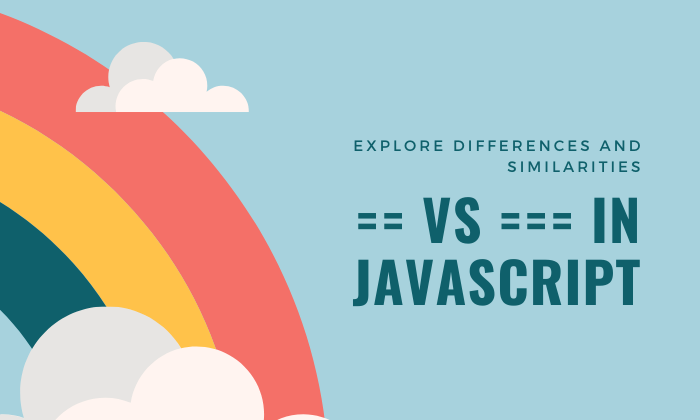## CodeNewbie Community 🌱# == VS ===

Difference Between these Equality Operator in JavaScript

# JavaScript has two equality Operators for comparing values, And Double Equality (==) called as Loose equality and Triple Equality know as the strictly equality Operators,

# Now the question is What is the difference between both the operator,

#The "==" double equality operators applies loosed typing when comparing value like below example I mentioned

#e.g

``````console.log("20"== 20); // true
``````

in the above example we are comparing String "20" which is in double quotes and number 20 As you are codenewbie you guy's i have at least knows what is string and what is number i am assuming you guys have knowledge about string and number if you dont let me know in comment section i will answer your all query

Both types are different but "==" equality operators says its same.. because it returned true in console

#Now the Question arrived why this is happened..

#Why?

In the "==" Operator they are comparing value but not type So Before comparing if the types are not the same one type is coerced to fit the other now what is mean by coerced: the coerced means to force somebody to do something, for example by threatening someone so now in that case comparing values the doubled quality operators threating to do certain thing like converting its type

Javascript would coerce one of the values from their existing type to the type that is compatible with the other the value will compared,

In the Previous Example javascript coerce the string to a number so "20" becomes and that becomes equal to the other 20,

Here are more example

``````console.log(0 == false); //true
console.log("" == false); //true
``````

These are the also cases of coercion false is coerced to number and the equivalent of false is 0 is equal to 0

An in empty string example "" is coerced to boolean which is false and false is equal to false

Now about the triple equal "===" is also called as strict equality operator value types are not forced to changes this operators compares both value and type Here are some Good Example

``````console.log("20" === 20)' //false
console.log( 0 === false)//false
console.log("" === "")// true
connsole.log(0 === 0)//true
console.log("hello" === false) // false
``````

It is recommended to use the strict operators "===" to avoid inconsistent that can happen when coercion happens

If You Learn Something New On this Post